# Abstract Witt ring

(diff) ← Older revision | Latest revision (diff) | Newer revision → (diff)
Two series of definitions, that of J. Kleinstein and A. Rosenberg [a1] and M. Marshall [a2], led to the same class of rings, which is now the most widely used. In this sense, an abstract Witt ring is a pairwhere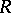is a commutative ring with unitandis a subgroup of the multiplicative groupwhich has exponent two, containsand generatesadditively. Let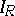denote the ideal ofgenerated by elements of the form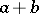, with. It is further assumed that:
1) if, then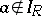;
2) ifand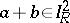, then;
3) if, withand all, then there exist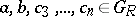such thatand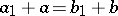.
Whenis the Witt ring of a field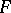, then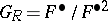and property 3) is a consequence of the Witt cancellation theorem.